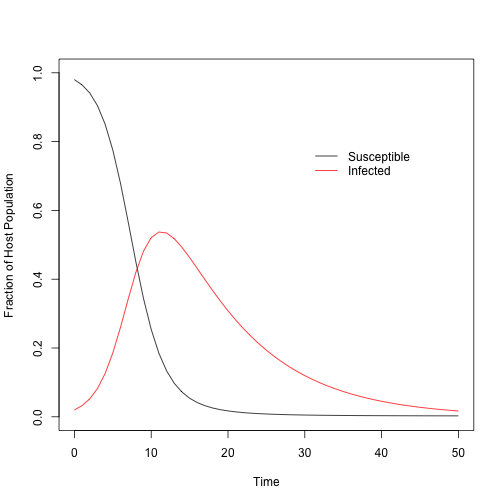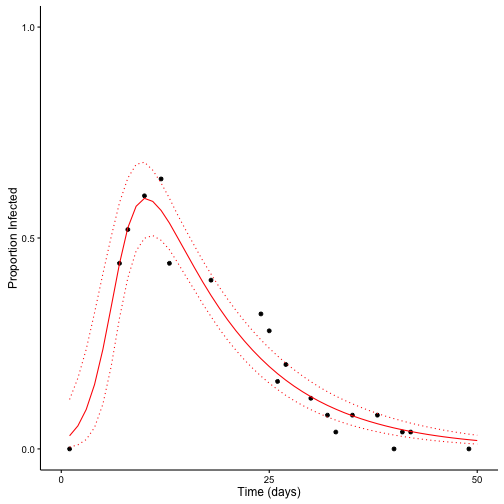Mechanistic disease models are at the heart of disease ecology and have generated fundamental biological insights, ranging from our understanding of disease-density thresholds to the influence of host heterogeneity on the spread of disease. The definition of a mechanistic model is actually debated quite a lot, but here I am referring specifically to systems of ordinary differential equations (ODEs) that can capture the important non-linearities and temporal dynamics of infection, which are founded on the idea of transmission from infectious hosts to susceptible hosts. This is probably the simplest example:

\begin{align} \frac{dS}{dt} & = - \beta S I \\ \frac{dI}{dt} & = \beta S I - \gamma I \\ \frac{dR}{dt} & = \gamma I \end{align}

In this simple $SIR$ model, $S$, $I$, and $R$ represent the fraction of susceptible, infected, and removed hosts, respectively, where $S + I + R = 1$. $\beta$ represents the frequency-dependent transmission, where $\beta S I$ informs the rate at which new individuals become infected, as a proportion of the total population. And, finally, $\gamma$ is the rate of death due to infection (i.e. virulence). In some models this is considered the recovery or immune rate. I’m assuming, among other things, that there is no recovery, only death due to infection.

Because mechanistic models like this one do such a good job at capturing the non-linear dynamics of infection, they are often used as predictive tools to inform, for instance, how vaccination or climate change or (insert your favorite meme) might influence disease epidemics. However, it is rare to see these models parameterized in rigorous ways, likely because this can involve complicated experiments that might be infeasible, say, in human systems. Moreover, non-linear model-fitting routines can be computationally costly and might lie outside the expertise of field ecologists who collect the necessary data.

However, if we collect our data in simple, yet calculated, ways, new technologies make model-fitting a surmountable challenge. And model-fitting can help get us those coveted parameter estimates that we need to make predictions. Furthermore, if model-fitting is done in a Bayesian framework, in cases where some parameters can be estimated with experimental data, we can combine information from the lab and from the field in a rigorous analysis. For instance, we can fit a mechanistic model to epidemic data collected from the field, and we can use experimental measurements of some or all model parameters to construct prior likelihoods.

In this blog post, I will show how we can fit a simple, mechanistic $SIR$ model to simulated data - representing easily collected data from the field - using R and Stan.

First, I will generate data by integrating an $SIR$ model with known $\beta$ and $\gamma$ parameters. This will simulate an epidemic window, which represents a time period over which data can be collected. For instance, we can go to the field and measure the proportion of hosts infected at various time points to capture the rise and fall of infection. This data is used to fit the model.The parameters represent a particularly virulent pathogen, where by the end of 50 days, most of the population has been infected and has died. This gives us the “true” epidemic dynamics of the system, from which we can simulate data that an ecologist might collect. For instance, an ecologist could go to the field and sample a given number of individuals from the population and figure out how many are infected. This could be repeated a number of times throughout the epidemic period, as follows:

Now that we have some synthetic data, let’s fit the mechanistic model using the Stan MCMC sampling software. I would highly recommend consulting the Stan documentation for fitting ODEs, but I’ll do my best to annotate the model statement. In the model, we’ll estimate the two parameters of interest $\beta$ and $\gamma$, as well as the initial conditions, which were likely unknown in the field.

Now we can validate the model. We’ll see how well our the Stan model fits to the synthetic data and we adequately estimate the known parameter values.The Stan model does a good job estimating the parameters and predicting unobserved data.

Admittedly, this “simple” approach will not work in some cases. For example, I cannot think of a good way to fit systems of stochastic differential equations in Stan, where the likelihood has to be averaged across many realizations of the system. Or, for models that have to estimate the number of, say, exposed or infected classes, which is an integer. Stan does not allow integer parameters, at least not yet. In my postdoc I am using custom MCMC scripts in C, which allows for fast integration with Monte MISER. This is certainly more challenging, though.

Updated: